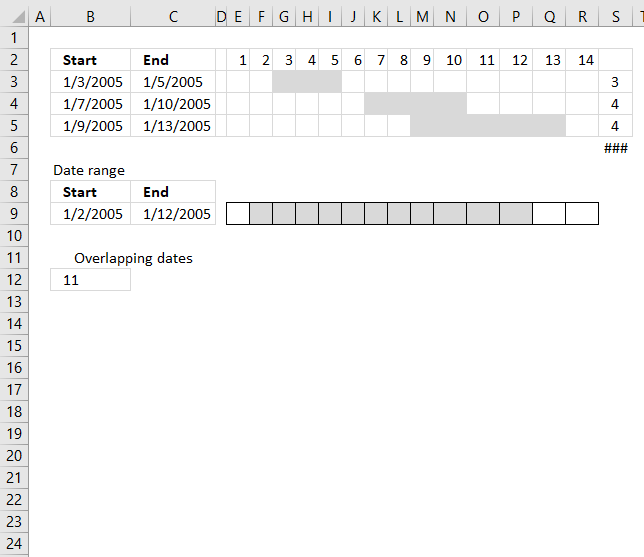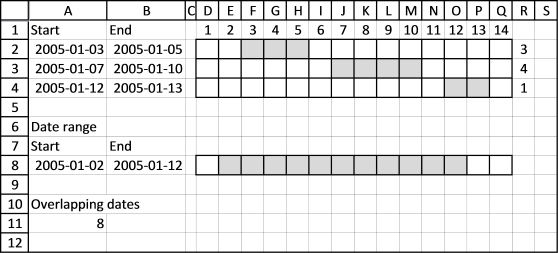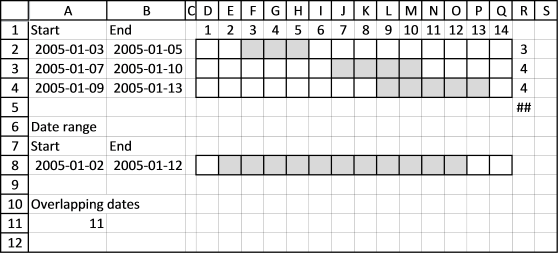Author: Oscar Cronquist Article last updated on October 09, 2018The MEDIAN function lets you count overlapping dates between two date ranges. If you have more than two date ranges you need to use a more complicated array formula.

I have three date ranges (A2:B4) in this example and I want to count the number of days that overlap another date range (A8:B8).This array formula counts overlapping days for each date range in cell range A2:B4 compared to date range in cell range A8:B8.

Array formula in cell R2:R4:

=MMULT((TRANSPOSE(\$A\$8+ROW(A1:INDEX(\$A:\$A, \$B\$8-\$A\$8+1))-1)>=\$A\$2:\$A\$4)*(TRANSPOSE(\$A\$8+ROW(A1:INDEX(\$A:\$A, \$B\$8-\$A\$8+1))-1)<=B2:B4), ROW(A1:INDEX(\$A:\$A, \$B\$8-\$A\$8+1))^0)

It returns this array: {3; 4; 1}. Date range 2005-01-03/2005-01-05 (A2:B2) has 3 overlapping dates compared to 2005-01-02/2005-01-12 (A8:B8). Date range 2005-01-07/2005-01-10 (A3:B3) has 4 overlapping dates compared to 2005-01-02/2005-01-12 (A8:B8). Date range 2005-01-12/2005-01-13 (A3:B3) has 1 overlapping date compared to 2005-01-02/2005-01-12 (A8:B8).

To count all overlapping days, array formula in cell A11:

=SUM(MMULT((TRANSPOSE(\$A\$8+ROW(A1:INDEX(\$A:\$A, \$B\$8-\$A\$8+1))-1)>=\$A\$2:\$A\$4)*(TRANSPOSE(\$A\$8+ROW(A1:INDEX(\$A:\$A, \$B\$8-\$A\$8+1))-1)<=B2:B4), ROW(A1:INDEX(\$A:\$A, \$B\$8-\$A\$8+1))^0))

Functions in array formulas: MMULT, ROW, INDEX

### Overlapping date ranges in cell range A2:B4

Keep in mind that if you have overlapping date ranges in A2:B4, overlapping dates will be counted twice or more.In this example, date 9 and 10 are overlapped by 2005-01-07/2005-01-10 and 2005-01-09/2005-01-13. They are counted twice, see cell range R3:R4.

The value in cell A11 is wrong because of this, only 9 dates are overlapped. There is a formula for this scenario also but I'll save it for a future post.